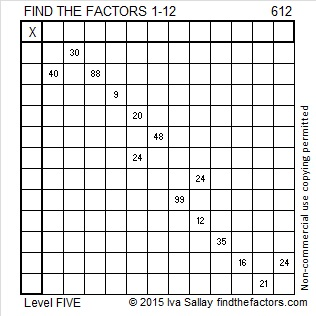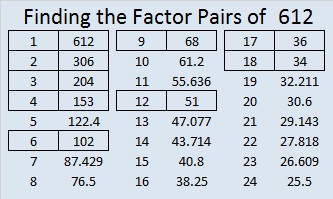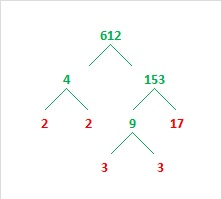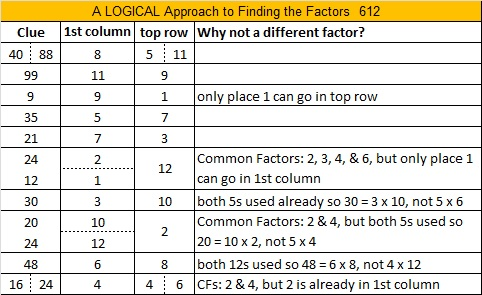# 612 and Level 5

612 is the hypotenuse of the Pythagorean triple 288-540-612. Which factor of 612 is the greatest common factor of those three numbers?

612 = 17 x 36, which is 17 x 18 x 2, and that is exactly four times the formula of the 17th triangular number. Thus . . .

612 = 4(1 + 2 + 3 + 4 + 5 + 6 + 7 + 8 + 9 + 10 + 11 + 12 + 13 + 14 + 15 + 16 + 17)

612 can be expressed as the sum of consecutive counting numbers in several ways:

• 612 = 203 + 204 + 205 (3 consecutive numbers because it is divisible by 3)
• 612 = 73 + 74 + 75 + 76 + 77 + 78 + 79 + 80 (eight consecutive numbers because it is divisible by 4, but not 8)
• 612 = 64 + 65 + 66 + 67 + 68 + 69 + 70 + 71 + 72 (9 consecutive numbers because it is divisible by 9)
• 612 = 28 + 29 + 30 + 31 + 32 + 33 + 34 + 35 + 36 + 37 + 38 + 39 + 40 + 41 + 42 + 43 + 44 (17 consecutive numbers because it is divisible by 17)
• 612 = 14 + 15 + 16 + 17 + . . . . + 34 + 35 + 36 + 37 (24 consecutive numbers because 612 is divisible by 12, but not by 24)

What is a relationship between the numbers in bold print and the number 612?

612 is also the sum of the twelve prime numbers from 29 to 73.Print the puzzles or type the solution on this excel file: 12 Factors 2015-09-07

—————————————————————————————————

• 612 is a composite number.
• Prime factorization: 612 = 2 x 2 x 3 x 3 x 17, which can be written 612 = (2^2) x (3^2) x 17
• The exponents in the prime factorization are 2, 2 and 1. Adding one to each and multiplying we get (2 + 1)(2 + 1)(1 + 1) = 3 x 3 x 2 = 18. Therefore 612 has exactly 18 factors.
• Factors of 612: 1, 2, 3, 4, 6, 9, 12, 17, 18, 34, 36, 51, 68, 102, 153, 204, 306, 612
• Factor pairs: 612 = 1 x 612, 2 x 306, 3 x 204, 4 x 153, 6 x 102, 9 x 68, 12 x 51, 17 x 36 or 18 x 34
• Taking the factor pair with the largest square number factor, we get √612 = (√36)(√17) = 6√17 ≈ 24.73863Although I prefer using a modified cake method to find square roots, most people prefer factor trees. If you use a factor tree, I suggest you still look for easy-to-detect perfect square factors (100, 4, 9, 25) so that the most common duplicate prime factors are together:—————————————————————————————————## 2 thoughts on “612 and Level 5”

1.Paula Beardell Krieg

Whew, seems like the number 612 has a great bit of commentary. As your numbers get bigger it sometimes seems like there’s less to say, but then for other numbers it seems just the opposite. I am in awe of how you are keeping this going. (thank you)

•ivasallay

You’re welcome! It makes me so happy that you enjoy reading about each number. Thank you for your interest. You are one of the reasons I want to keep this going.

This site uses Akismet to reduce spam. Learn how your comment data is processed.